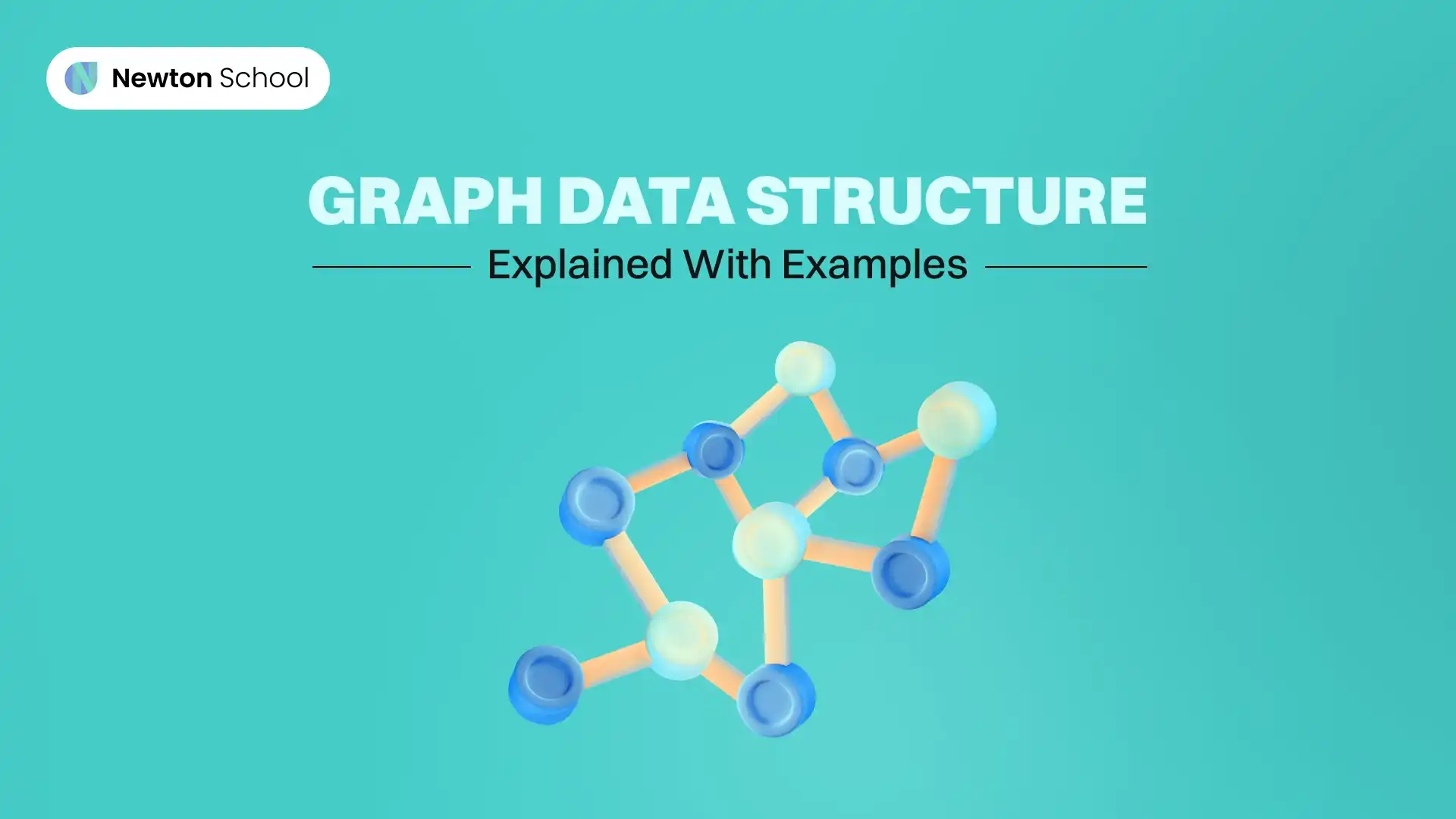# Graph Data Structure - Explained With Examples

July 28, 2023A graph data structure presents a pictorial way of connecting nodes through links. From technical subject books in engineering to real-world applications, these non-linear data structures are ubiquitous. In computer science, graph data structures illustrate the sequence of computation. On the other hand, applications like Facebook use graphs to create a link (connection) between two vertices (profiles of two individuals). Despite their omnipresence, the technical implementation of this concept can pose difficulties if they aren't understood from scratch.

## Define Graph In Data Structure

In a broader sense, data structures are categorised as linear and non-linear. Stacks, queues, and linked lists are types of linear structures. On the contrary, trees and graphs constitute non-linear structures. To understand graphs, you must first become familiar with the basic terms used to explain this concept. These are:

• Nodes: If you visually imagine a graph, the nodes are the vertices in that image. For example, in a computer lab with computers connected to the internet through Ethernet cable, each computer is a node connected to a centralised LAN.
• Edges: Edges are the link that connects every node to a centralised location. This centralised location could be local or global. For example, if two computers are connected to a centralised server through a cable wire, the cable wire is the edge, and the server is a centralised global location. However, suppose you add another computer connected to the server and connect this computer to two other computers. In that case, the new two computers are not connected to the server directly but through the third computer. The third computer is the local centralised location for the last two computers.

As you might have already understood, a graph is a collection of a finite number of nodes connected through a finite number of edges. Technically, a graph is represented as a set of vertices and edges. The vertices and edges of the graph in the image below are:

V= {1,2,3,4,5}

E= {(1,2), (1,3), (2,3), (2,4), (2,5), (3,2), (3.5), (4,5)}

As you can notice, the edges that have been repeated are only mentioned once in the set. For instance, out of (2,4) and (4,2), only one edge has been listed.

• Path: A path is a sequence of edges that allows you to go from one vertex to another. For instance, 1-2 and 1-3-2 paths from vertex 1 to vertex 2.
• Adjacency: Two vertices are said to be adjacent when an edge connects them. In this example, vertex 3 and 4 are not adjacent as an edge doesn't connect them.

## Types Of Graph In Data Structure

Listed below are the common types of graphs in the data structure.

• Null: A graph with only vertices and no edges is called a null graph.
• Trivial: A rival graph is the one with no boundaries and only one vertex.
• Finite: A graph with a limited number of vertices and edges is called a finite graph.
• Infinite: A graph with an endless number of vertices and edges is called an infinite graph.
• Digraph: Also known as a directed graph, a digraph contains a pair of vertices (V1, V2) for every edge.

## Representation of Graphs

We have explained the two general ways of representing graphs in the following sub-sections.

• Adjacency Matrix: Create a 2-dimensional array of Vertices x Vertices (VxV) size. For example, let us consider a 2x2 matrix for a graph with two vertices. The matrix would look like the image below. Here, 0 and 1 are used to represent the edges between vertices. As elements 1x2 and 2x1 are 1, vertices 2 and 1 are connected by an edge.
• NOTE FOR THE EDITOR: The image is self-made through Google Docs. Thus, there is no link for reference.
• Adjacency List: As the name says, an adjacency list uses a linked list for showing the connection between the vertices and edges of a graph. For instance, a chart connects vertices 1,2 and 3 with edges as (1,2), (2,3), AND (3,1). As shown below, there would be three linked lists with elements and their links.
• 1-2-3
• 2-3
• 3-1

## Applications of Graph Data Structure

The edges of a graph can be of two types: directed and undirected. In a directed graph, the boundaries are drawn using an arrow from one vertex to another. On the contrary, an undirected graph uses straight lines (with no direction) to join one vertex to another. The applications of such a graph data structure are listed below.

• World Wide Web: The Google Page Ranking Algorithm leveraged the directed graph structure of the world wide web. It ranked pages with the highest number of links (edges between the vertices of websites) at the top.
• Google Maps: Algorithms like the Dijkstra algorithm find the shortest distance between two vertices in a graph. Such algorithms are used in Google Maps for calculating the estimated distance and time between two points on the map (vertices).
• Social Media: Social media platforms use graphs heavily for suggesting friends, posts, reels, and pages for you to follow. These suggestions act as individual vertices connected to your profile's vertex.

## Learn Graph Data Structure online

Newton School offers professional courses for graduates looking for high-paying job opportunities. You can learn about graph data structures online by taking one of the many courses our professionals provide. With over 800 hiring partners in our network, you can get the job you desire immediately after completing the course.